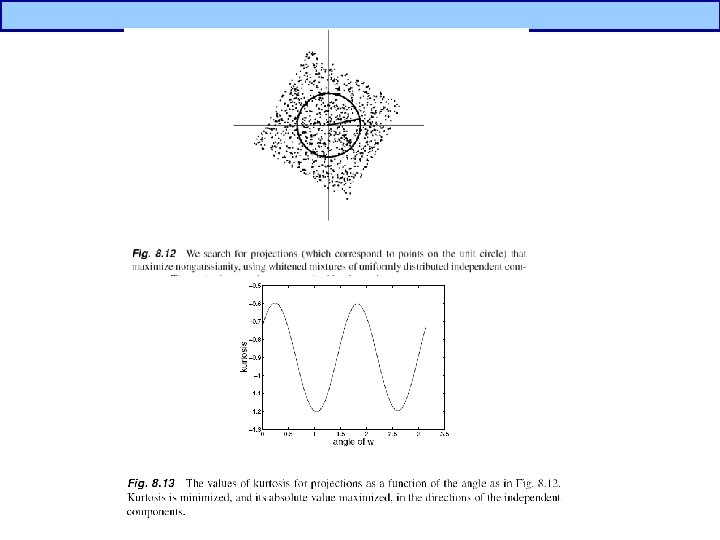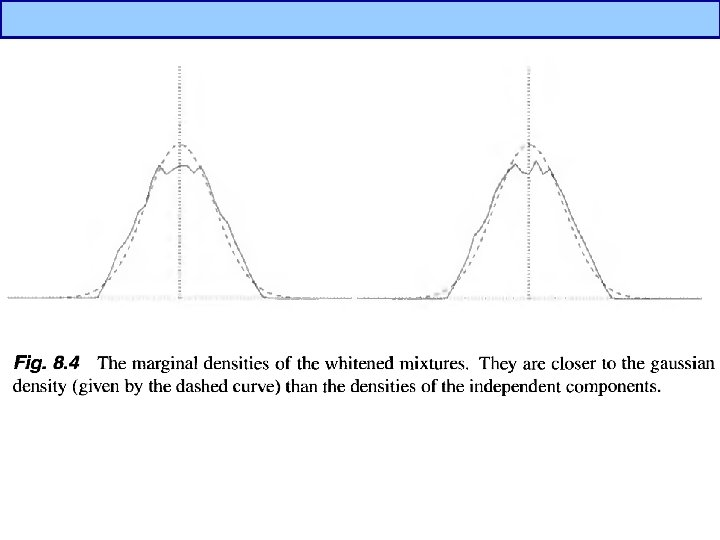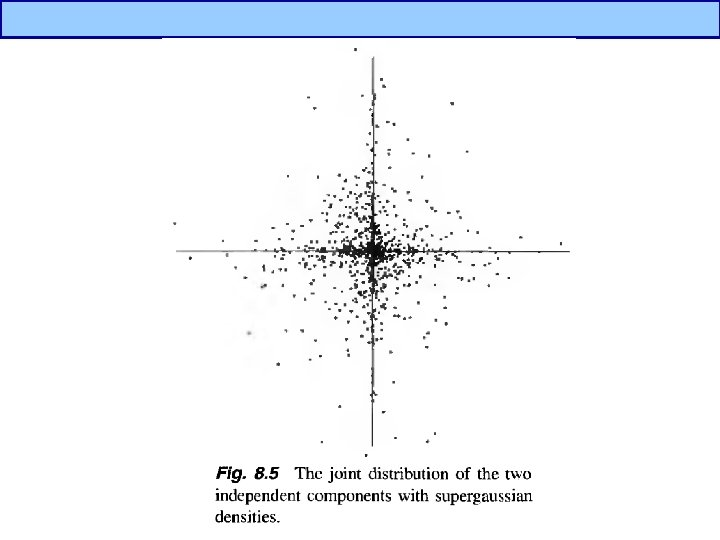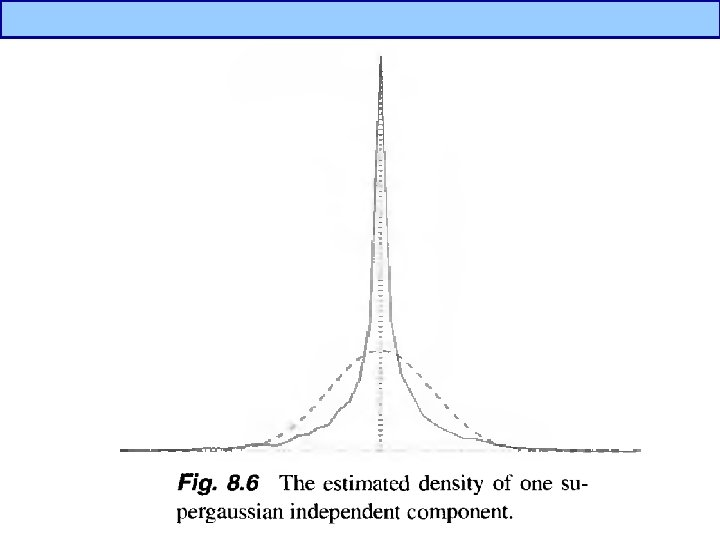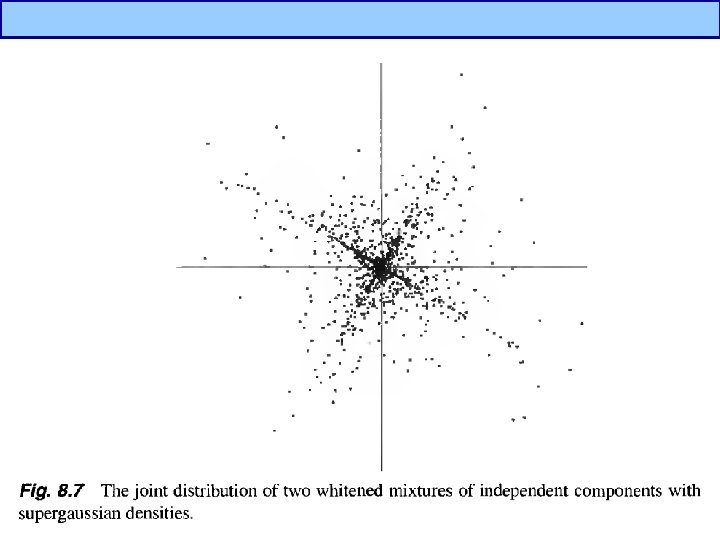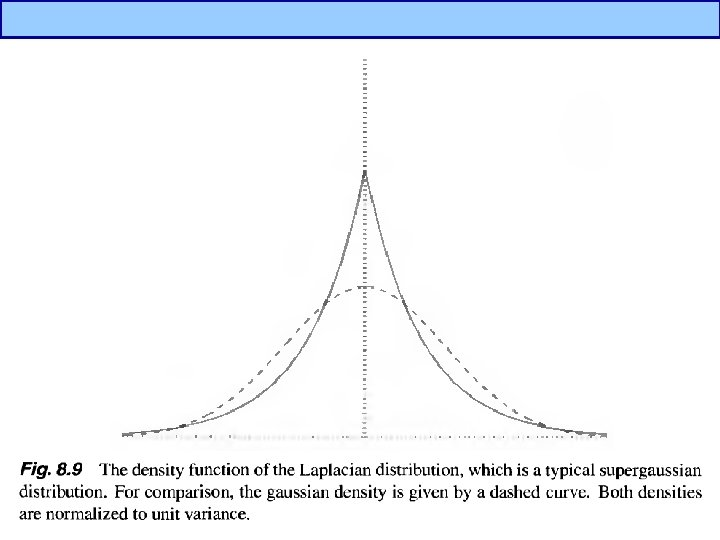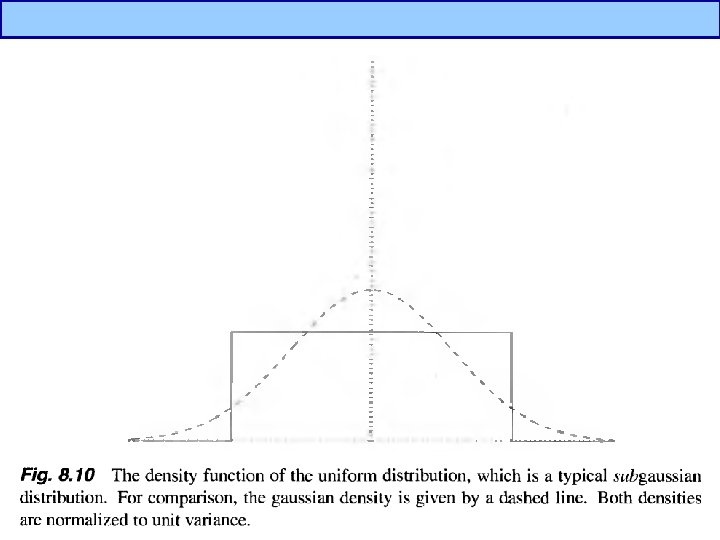# PCA vs ICA PCA Focus on uncorrelated and

• Slides: 20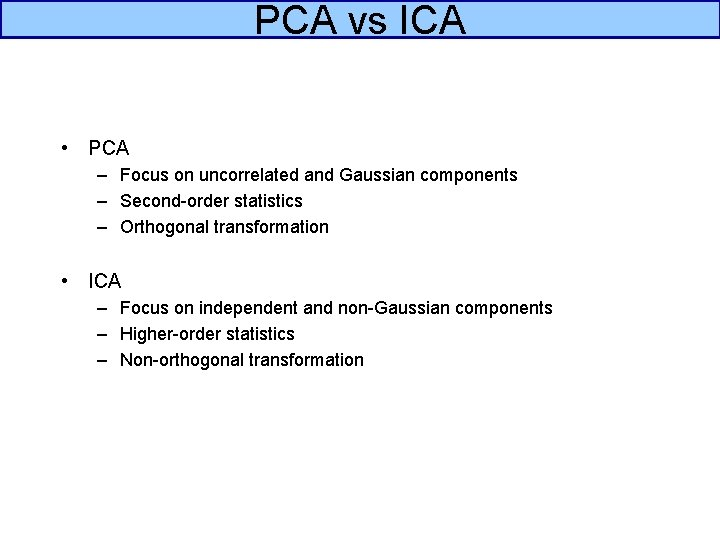PCA vs ICA • PCA – Focus on uncorrelated and Gaussian components – Second-order statistics – Orthogonal transformation • ICA – Focus on independent and non-Gaussian components – Higher-order statistics – Non-orthogonal transformation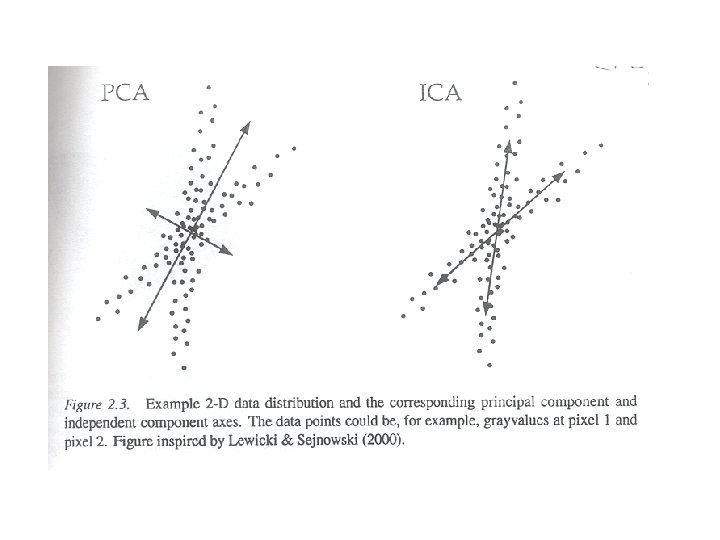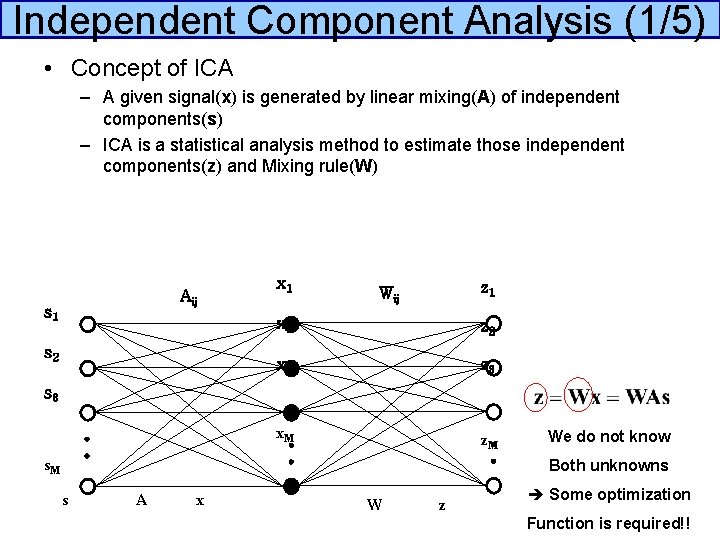Independent Component Analysis (1/5) • Concept of ICA – A given signal(x) is generated by linear mixing(A) of independent components(s) – ICA is a statistical analysis method to estimate those independent components(z) and Mixing rule(W) Aij s 1 s 2 x 1 z 1 Wij x 2 z 2 x 3 z 3 x. M z. M s 3 s. M We do not know Both unknowns s A x W z Some optimization Function is required!!Independent Component Analysis (2/5)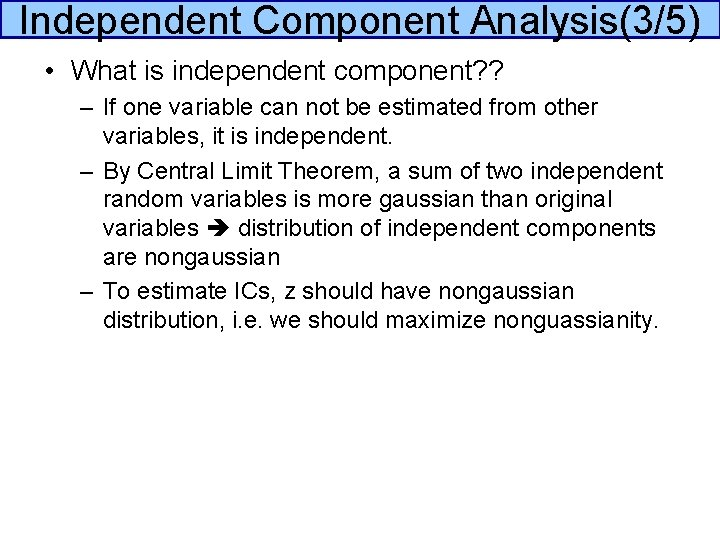Independent Component Analysis(3/5) • What is independent component? ? – If one variable can not be estimated from other variables, it is independent. – By Central Limit Theorem, a sum of two independent random variables is more gaussian than original variables distribution of independent components are nongaussian – To estimate ICs, z should have nongaussian distribution, i. e. we should maximize nonguassianity.Independent Component Analysis(4/5) • What is nongaussianity? – Supergaussian – Subgaussian – Low entropy Gaussian Supergaussian Subgaussian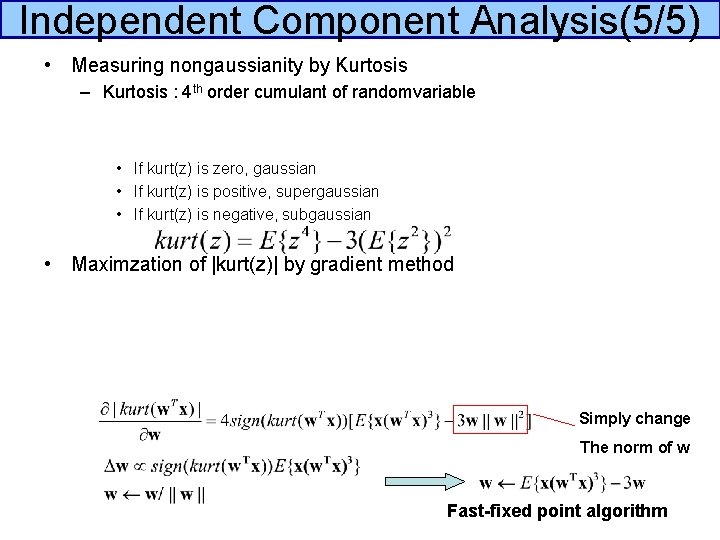Independent Component Analysis(5/5) • Measuring nongaussianity by Kurtosis – Kurtosis : 4 th order cumulant of randomvariable • If kurt(z) is zero, gaussian • If kurt(z) is positive, supergaussian • If kurt(z) is negative, subgaussian • Maximzation of |kurt(z)| by gradient method Simply change The norm of w Fast-fixed point algorithmPCA vs LDA vs ICA • PCA : Proper to dimension reduction • LDA : Proper to pattern classification if the number of training samples of each class are large • ICA : Proper to blind source separation or classification using ICs when class id of training data is not available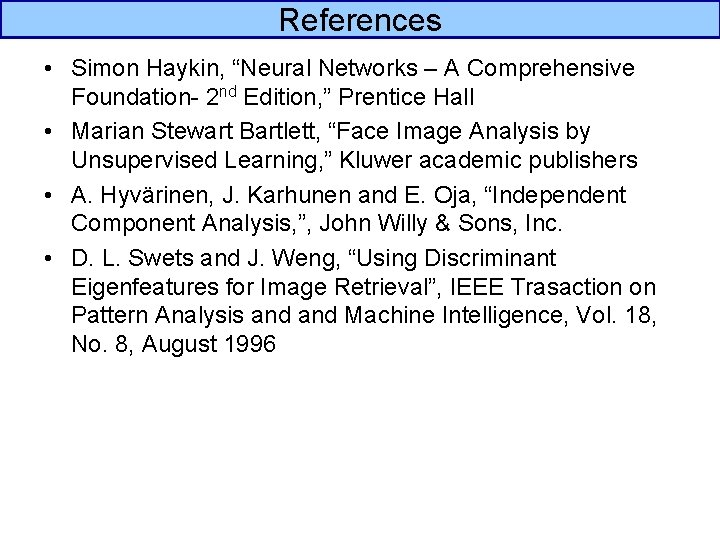References • Simon Haykin, “Neural Networks – A Comprehensive Foundation- 2 nd Edition, ” Prentice Hall • Marian Stewart Bartlett, “Face Image Analysis by Unsupervised Learning, ” Kluwer academic publishers • A. Hyvärinen, J. Karhunen and E. Oja, “Independent Component Analysis, ”, John Willy & Sons, Inc. • D. L. Swets and J. Weng, “Using Discriminant Eigenfeatures for Image Retrieval”, IEEE Trasaction on Pattern Analysis and Machine Intelligence, Vol. 18, No. 8, August 1996